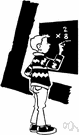# multiplication

(redirected from Integer multiplication)
Also found in: Thesaurus, Medical, Encyclopedia.

## mul·ti·pli·ca·tion

(mŭl′tə-plĭ-kā′shən)
n.
1. The act or process of multiplying or the condition of being multiplied.
2. Propagation of plants and animals; procreation.
3. Mathematics
a. The operation that, for positive integers, consists of adding a number (the multiplicand) to itself a certain number of times. The operation is extended to other numbers according to the multiplicative properties of positive integers and other algebraic properties.
b. Any of certain analogous operations involving mathematical objects other than numbers.

## multiplication

(ˌmʌltɪplɪˈkeɪʃən)
n
1. (Mathematics) an arithmetical operation, defined initially in terms of repeated addition, usually written a × b, a.b, or ab, by which the product of two quantities is calculated: to multiply a by positive integral b is to add a to itself b times. Multiplication by fractions can then be defined in the light of the associative and commutative properties; multiplication by 1/n is equivalent to multiplication by 1 followed by division by n: for example 0.3 × 0.7 = 0.3 × = (0.3 × 7)/10 = 2 = 0.21
2. the act of multiplying or state of being multiplied
3. (Biology) the act or process in animals, plants, or people of reproducing or breeding

## mul•ti•pli•ca•tion

(ˌmʌl tə plɪˈkeɪ ʃən)

n.
1. the act or process of multiplying or the state of being multiplied.
2. a mathematical operation, symbolized by axb, a b, a * b, or ab, and signifying, when a and b are positive integers, that a is to be added to itself as many times as there are units in b; the addition of a number to itself as often as is indicated by another number, as in 2x3 or 5x10.
3. any generalization of this operation applicable to numbers other than integers, as fractions or irrational numbers.
[1350–1400; Middle English < Latin]

## mul·ti·pli·ca·tion

(mŭl′tə-plĭ-kā′shən)
1. The mathematical operation that consists of adding a number (the multiplicand) to itself a specified number of times. Thus multiplying 6 by 3 means adding 6 to itself three times.
2. A mathematical operation performed on a pair of numbers in order to derive a third number called a product. It is sometimes convenient to consider multiplication as repeated addition in which one number indicates how many times the other is to be added together. For example, 3 × 4 = 4 + 4 + 4 = 4 × 3 = 3 + 3 + 3 + 3 = 12.
ThesaurusAntonymsRelated WordsSynonymsLegend:
 Noun 1 multiplication - the act of producing offspring or multiplying by such productionfacts of life, procreation, reproduction, breeding - the sexual activity of conceiving and bearing offspringbiogeny, biogenesis - the production of living organisms from other living organisms 2 multiplication - a multiplicative increase; "repeated copying leads to a multiplication of errors"; "this multiplication of cells is a natural correlate of growth"doubling - increase by a factor of two; "doubling with a computer took no time at all"increment, growth, increase - a process of becoming larger or longer or more numerous or more important; "the increase in unemployment"; "the growth of population"quadrupling - increase by a factor of fourquintupling - increasing by a factor of fivetripling - increase by a factor of three 3multiplication - an arithmetic operation that is the inverse of division; the product of two numbers is computed; "the multiplication of four by three gives twelve"; "four times three equals twelve"timesarithmetic operation - a mathematical operation involving numbers

## multiplication

noun
1. The act of increasing or rising:
2. The result or product of building up:
3. The process by which an organism produces others of its kind:
Obsolete: increase.
Translations
تَكاثُرضَرْبُ
násobení
multiplikation
razmnožavanje
szorzás
margföldun

곱셈
násobenie
množenje
multiplikation
การคูณ
çarpım işlemiçarpmaçarpma işlemi
sự nhân

## multiplication

[ˌmʌltɪplɪˈkeɪʃən]
A. N
B. CPD multiplication table N

## multiplication

[ˌmʌltɪplɪˈkeɪʃən] n
(MATHEMATICS) [numbers] →
[cells, bacteria] →
(= increase) [things] →
the multiplication of political crises → multiplication sign nmultiplication table n

## multiplication

n
(Math) → Multiplikation f
(fig)Vervielfachung f, → Vermehrung f

## multiplication

:
multiplication sign
n (Math) → Multiplikationszeichen nt
multiplication table
n (Math) → Multiplikationstabelle f; he knows all his multiplicationser kann das Einmaleins

## multiplication

[ˌmʌltɪplɪˈkeɪʃn] n

## multiply

(ˈmaltiplai) verb
1. to add a number to itself a given number of times and find the total. 4 + 4 + 4 or 4 multiplied by 3 or 4  3 = 12.
2. to (cause to) increase in number, especially by breeding. Rabbits multiply very rapidly.
ˌmultipliˈcation (-pli-) noun
the act of multiplying numbers.

## multiplication

násobení multiplikation kertolasku razmnožavanje 掛け算 곱셈 multiplikation การคูณ sự nhân
References in periodicals archive ?
Furer, "Faster integer multiplication," SIAM Journal on Computing, vol.
Hence, the effect of optimizations like replacing multiplication by a multiplier which is a power of two (an example of integer multiplication) by left shifting the multiplicand variable has already been considered.
 Jebelean, T., "Using the parallel karatsuba algorithm for long integer multiplication and division," in Proc.

Site: Follow: Share:
Open / Close